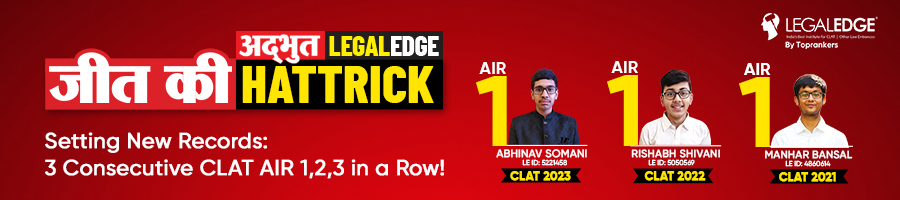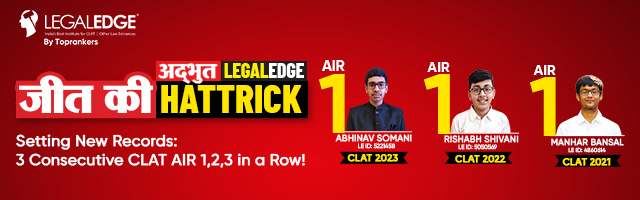# CLAT Maths Syllabus 2024: Key Topics & Important Sections

Author : Tanya Kaushal

Updated On : November 22, 2023

SHARE

Reader's Digest: Hey there! Wondering about the CLAT 2024 Maths Syllabus? Well, let us give you a sneak peek! So, let's dive into the CLAT Maths Syllabus 2024 and prepare for the big day, shall we?

The Quantitative Techniques syllabus for CLAT 2024 focuses on understanding and using numerical and visual data and basic math concepts from the 10th grade.

The Maths CLAT syllabus includes short information presented in graphs, charts, and diagrams, followed by questions that require mathematical operations.

Mathematical operations must be performed while applying mathematical concepts. The information provided forms the foundation for interpretation and must be processed to answer the question.

The Quantitative Techniques section is considered one of the highest-scoring areas in CLAT, and a thorough understanding of the CLAT Mathematics Syllabus 2024 can significantly improve your overall CLAT rank.

Here is a glimpse of the main points that will be discussed in the blog:

1. Official CLAT Quant Syllabus by Consortium: Understanding the core topics you must master.
2. Prep Strategies to Ace CLAT Maths Syllabus: Expert CLAT Maths preparation tips for effective preparation strategy.
3. Type of Questions Asked in the CLAT: A breakdown of question types and patterns as per the CLAT quantitative aptitude syllabus.
4. Top Books to Cover CLAT Maths Syllabus 2024: Recommended study resources to excel in the CLAT exam

Here is a video by Legal Edge going through several Quantitative Techniques questions from the Maths syllabus in CLAT, which are going to be extremely helpful in navigating this section:

### Overview of CLAT Maths Syllabus 2024

In the Quantitative Techniques section of the CLAT UG syllabus, you will encounter short sets of facts or propositions, graphs, and different ways of representing numerical information through text, pictures, or diagrams.

This section tests your ability to extract important information from these passages, graphs, or representations and use mathematical operations to analyze and solve the questions provided.

The purpose of this section is to evaluate your Quantitative Techniques and problem-solving skills within the context of legal education by applying mathematical concepts to real-life situations.

## Official CLAT Maths Syllabus 2024

The CLAT exam 2024 will have a section called "Quantitative Reasoning." In this section, you will be given tasks that involve:

1. Understanding and using numerical information in passages, graphs, or other formats.
2. Using basic math operations like ratios, proportions, algebra, measurement, and statistics based on your knowledge in 10th grade.

These questions test how well you can analyze and work with numbers. You need to have a good grasp of math concepts and know how to apply them to do well. Make a Quantitative Techniques Study Plan for CLAT 2024.## Type of Questions Asked in the CLAT Maths Syllabus 2024

You are given information that needs to be read and understood for the Quantitative Techniques section. After reading the information, you have to answer the related questions.

You can come across different topic-based questions in one passage. In the CLAT Question Paper, the mathematics section includes data interpretation-based questions.

Here are some important topics from the CLAT Maths Syllabus 2024 that you should pay special attention to:

• Ratio and Proportion: Understanding ratios and proportions are crucial for solving various mathematical problems. It includes topics like direct and inverse proportions, comparison of ratios, and solving problems based on proportions.
• Basic Algebra: Questions involving algebraic expressions, linear and quadratic equations, and arithmetic progression.
• Mensuration: Problems related to calculating the area, volume, and surface area of geometric shapes.
• Statistical Estimation: Questions on data interpretation, including mean, median, mode, and other statistical concepts.

By dedicating sufficient time and effort to these important topics, you can strengthen your grasp of the CLAT Maths Syllabus 2024 and improve your performance in the exam.

### Effective Preparation Strategies for CLAT Maths Syllabus 2024

Solving CLAT Maths Questions requires a structured approach to ensure thorough understanding and efficient problem-solving.

Here are some effective preparation strategies to enhance your performance in the CLAT Quantitative Techniques section:

1. Understand the Fundamentals: Revisiting the fundamental mathematical concepts from the 10th-grade syllabus. Build a strong foundation in arithmetic, algebra, geometry, and trigonometry.
2. Learn Problem-Solving Techniques: Familiarize yourself with different problem-solving techniques and strategies for each topic. Practice applying these techniques to solve a variety of questions.
3. Practice Regularly: Consistent practice is key to improving your mathematical skills. Solve many practice questions, mock tests, and previous years' question papers to develop speed and accuracy.
4. Time Management: Develop effective time management skills to allocate appropriate time to each question during the exam. Practice solving questions within a specified time frame to enhance your speed.
5. Analyze and Learn from Mistakes: Review and analyze your mistakes while solving practice questions. Identify the areas where you face challenges and work on improving them through targeted practice.
6. Seek Clarification: If you encounter doubts or difficulties while studying or solving practice questions, don't hesitate to seek clarification from teachers, mentors, or online resources.
7. Mock Tests and Simulated Exams: Regularly take mock and simulated exams to assess your progress and get accustomed to the exam's pattern and time constraints.

Remember, consistent effort and dedication are crucial for success in the CLAT Maths section. Following these preparation strategies and focusing on the important topics can enhance your understanding and performance in the CLAT exam.### Recommended Books to Cover CLAT Maths Syllabus 2024

You can refer to the following books while preparing for CLAT Maths Syllabus 2024. Opting for the best books for CLAT will help you cover all the topics as per the latest syllabus.

 Book name Author/Publisher GMAT Official Guide Graduate Management Admission Council Modern Approach to Verbal and Non-Verbal Reasoning R.S Aggarwal Magical Book Series Data Interpretation BSC Publication

### Conclusion

Here are some important points about the CLAT 2024 Maths Syllabus:

1. The maths syllabus for CLAT is based on what you learn in the 10th grade.
2. The CLAT Maths Syllabus 2024 tests your understanding and application of basic math concepts.
3. It's important to practice and get familiar with different types of math questions.
4. Regularly reviewing and revising math concepts is necessary to remember them.

To do well in the math section of the CLAT Entrance Exam, it's crucial to have a good understanding of the CLAT Maths Syllabus 2024 and practice consistently.How can I better prepare for CLAT's Quantitative Techniques section?What are the best books for the preparation of the CLAT's Quantitative Techniques section?Do I need to take specific coaching for CLAT Maths?How many questions will be asked in the Quantitative Techniques section of CLAT?What is the difficulty level of Quantitative Techniques section in CLAT?Is 1 month preparation enough for CLAT Maths Preparation?